# Algorithmic Data Science = Theory + Practice

Jul. 23, 2018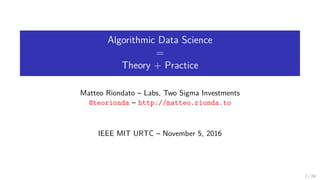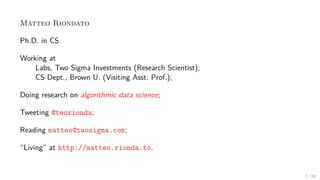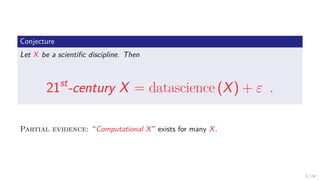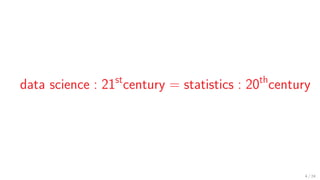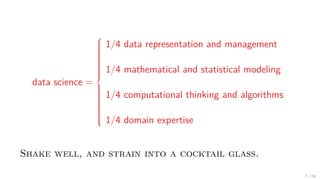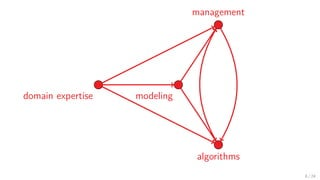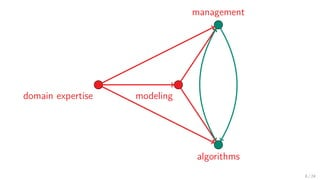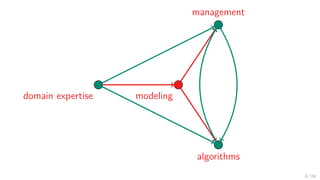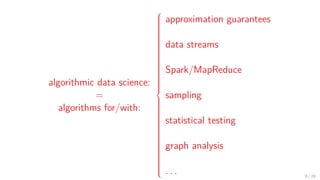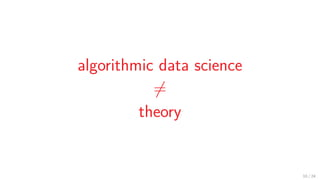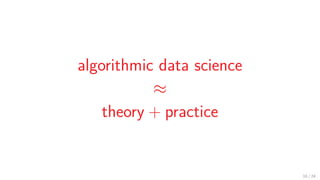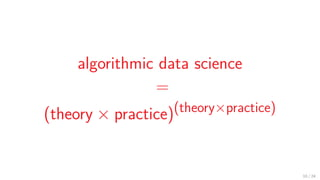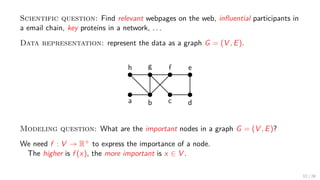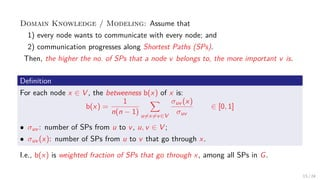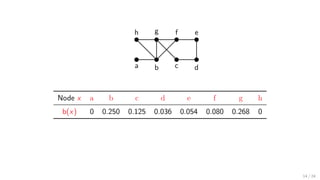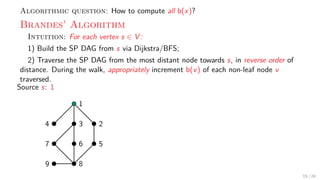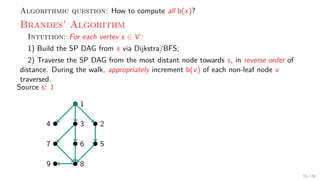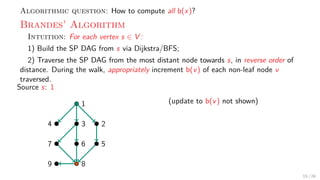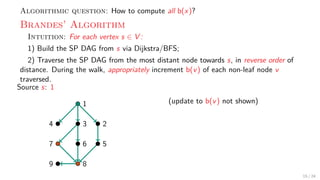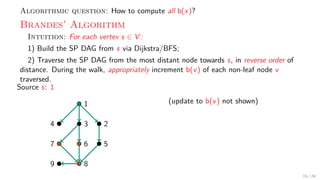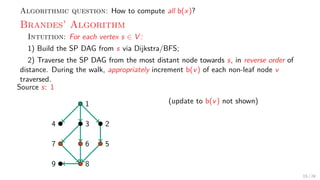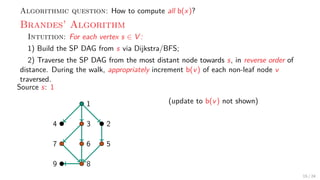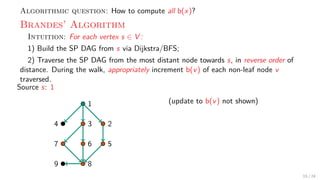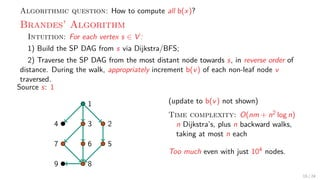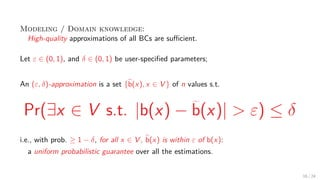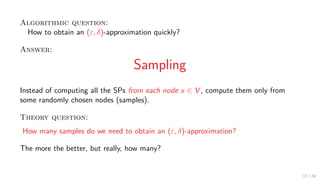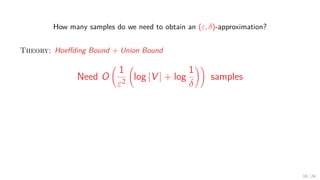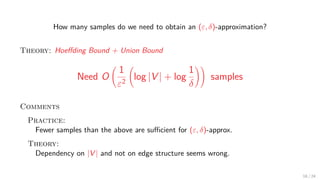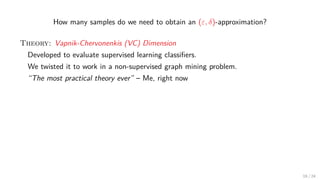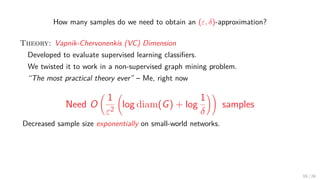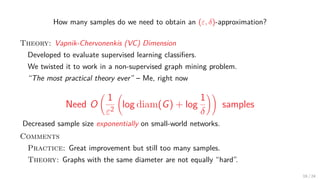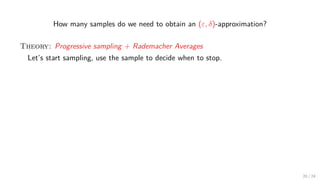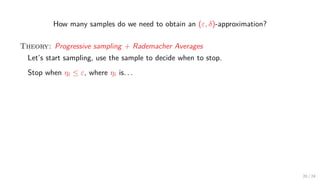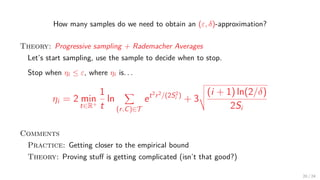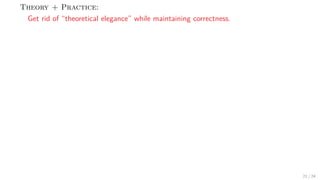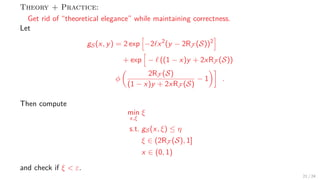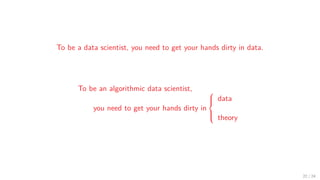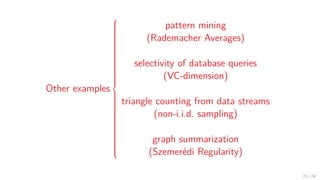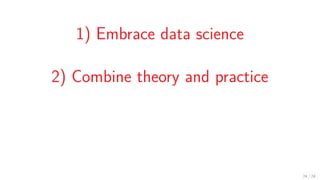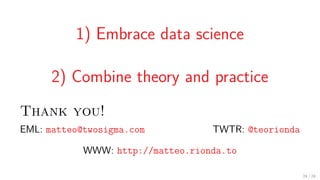1 of 48

### Algorithmic Data Science = Theory + Practice

• 1. Algorithmic Data Science = Theory + Practice Matteo Riondato – Labs, Two Sigma Investments @teorionda – http://matteo.rionda.to IEEE MIT URTC – November 5, 2016 1 / 24
• 2. Matteo Riondato Ph.D. in CS Working at Labs, Two Sigma Investments (Research Scientist); CS Dept., Brown U. (Visiting Asst. Prof.); Doing research on algorithmic data science; Tweeting @teorionda; Reading matteo@twosigma.com; “Living” at http://matteo.rionda.to. 2 / 24
• 3. Conjecture Let X be a scientiﬁc discipline. Then 21st -century X = datascience (X) + ε . Partial evidence: “Computational X” exists for many X. 3 / 24
• 4. data science : 21st century = statistics : 20th century 4 / 24
• 5. data science for 21st century society    questions data 5 / 24
• 8. data science =    1/4 data representation and management 1/4 mathematical and statistical modeling 1/4 computational thinking and algorithms 1/4 domain expertise Shake well, and strain into a cocktail glass. 7 / 24
• 12. algorithmic data science: = algorithms for/with:    approximation guarantees data streams Spark/MapReduce sampling statistical testing graph analysis . . . 9 / 24
• 15. algorithmic data science = (theory × practice)(theory×practice) 10 / 24
• 17. Scientific question: Find relevant webpages on the web, inﬂuential participants in a email chain, key proteins in a network, . . . Data representation: represent the data as a graph G = (V , E). a h b g f e c d Modeling question: What are the important nodes in a graph G = (V , E)? We need f : V → R+ to express the importance of a node. The higher is f (x), the more important is x ∈ V . 12 / 24
• 18. Domain Knowledge / Modeling: Assume that 1) every node wants to communicate with every node; and 2) communication progresses along Shortest Paths (SPs). Then, the higher the no. of SPs that a node v belongs to, the more important v is. Deﬁnition For each node x ∈ V , the betweeness b(x) of x is: b(x) = 1 n(n − 1) u=x=v∈V σuv (x) σuv ∈ [0, 1] • σuv : number of SPs from u to v, u, v ∈ V ; • σuv (x): number of SPs from u to v that go through x. I.e., b(x) is weighted fraction of SPs that go through x, among all SPs in G. 13 / 24
• 19. a h b g f e c d Node x a b c d e f g h b(x) 0 0.250 0.125 0.036 0.054 0.080 0.268 0 14 / 24
• 20. Algorithmic question: How to compute all b(x)? 15 / 24
• 21. Algorithmic question: How to compute all b(x)? Brandes’ Algorithm Intuition: For each vertex s ∈ V : 1) Build the SP DAG from s via Dijkstra/BFS; 2) Traverse the SP DAG from the most distant node towards s, in reverse order of distance. During the walk, appropriately increment b(v) of each non-leaf node v traversed. Source s: 1 1 234 567 89 15 / 24
• 22. Algorithmic question: How to compute all b(x)? Brandes’ Algorithm Intuition: For each vertex s ∈ V : 1) Build the SP DAG from s via Dijkstra/BFS; 2) Traverse the SP DAG from the most distant node towards s, in reverse order of distance. During the walk, appropriately increment b(v) of each non-leaf node v traversed. Source s: 1 1 234 567 89 15 / 24
• 23. Algorithmic question: How to compute all b(x)? Brandes’ Algorithm Intuition: For each vertex s ∈ V : 1) Build the SP DAG from s via Dijkstra/BFS; 2) Traverse the SP DAG from the most distant node towards s, in reverse order of distance. During the walk, appropriately increment b(v) of each non-leaf node v traversed. Source s: 1 1 234 567 89 (update to b(v) not shown) 15 / 24
• 24. Algorithmic question: How to compute all b(x)? Brandes’ Algorithm Intuition: For each vertex s ∈ V : 1) Build the SP DAG from s via Dijkstra/BFS; 2) Traverse the SP DAG from the most distant node towards s, in reverse order of distance. During the walk, appropriately increment b(v) of each non-leaf node v traversed. Source s: 1 1 234 567 89 (update to b(v) not shown) 15 / 24
• 25. Algorithmic question: How to compute all b(x)? Brandes’ Algorithm Intuition: For each vertex s ∈ V : 1) Build the SP DAG from s via Dijkstra/BFS; 2) Traverse the SP DAG from the most distant node towards s, in reverse order of distance. During the walk, appropriately increment b(v) of each non-leaf node v traversed. Source s: 1 1 234 567 89 (update to b(v) not shown) 15 / 24
• 26. Algorithmic question: How to compute all b(x)? Brandes’ Algorithm Intuition: For each vertex s ∈ V : 1) Build the SP DAG from s via Dijkstra/BFS; 2) Traverse the SP DAG from the most distant node towards s, in reverse order of distance. During the walk, appropriately increment b(v) of each non-leaf node v traversed. Source s: 1 1 234 567 89 (update to b(v) not shown) 15 / 24
• 27. Algorithmic question: How to compute all b(x)? Brandes’ Algorithm Intuition: For each vertex s ∈ V : 1) Build the SP DAG from s via Dijkstra/BFS; 2) Traverse the SP DAG from the most distant node towards s, in reverse order of distance. During the walk, appropriately increment b(v) of each non-leaf node v traversed. Source s: 1 1 234 567 89 (update to b(v) not shown) 15 / 24
• 28. Algorithmic question: How to compute all b(x)? Brandes’ Algorithm Intuition: For each vertex s ∈ V : 1) Build the SP DAG from s via Dijkstra/BFS; 2) Traverse the SP DAG from the most distant node towards s, in reverse order of distance. During the walk, appropriately increment b(v) of each non-leaf node v traversed. Source s: 1 1 234 567 89 (update to b(v) not shown) 15 / 24
• 29. Algorithmic question: How to compute all b(x)? Brandes’ Algorithm Intuition: For each vertex s ∈ V : 1) Build the SP DAG from s via Dijkstra/BFS; 2) Traverse the SP DAG from the most distant node towards s, in reverse order of distance. During the walk, appropriately increment b(v) of each non-leaf node v traversed. Source s: 1 1 234 567 89 (update to b(v) not shown) Time complexity: O(nm + n2 log n) n Dijkstra’s, plus n backward walks, taking at most n each Too much even with just 104 nodes. 15 / 24
• 30. Modeling / Domain knowledge: High-quality approximations of all BCs are suﬃcient. 16 / 24
• 31. Modeling / Domain knowledge: High-quality approximations of all BCs are suﬃcient. Let ε ∈ (0, 1), and δ ∈ (0, 1) be user-speciﬁed parameters; An (ε, δ)-approximation is a set {b(x), x ∈ V } of n values s.t. Pr(∃x ∈ V s.t. |b(x) − b(x)| > ε) ≤ δ i.e., with prob. ≥ 1 − δ, for all x ∈ V , b(x) is within ε of b(x): a uniform probabilistic guarantee over all the estimations. 16 / 24
• 32. Algorithmic question: How to obtain an (ε, δ)-approximation quickly? Answer: Sampling Instead of computing all the SPs from each node x ∈ V , compute them only from some randomly chosen nodes (samples). Theory question: How many samples do we need to obtain an (ε, δ)-approximation? The more the better, but really, how many? 17 / 24
• 33. How many samples do we need to obtain an (ε, δ)-approximation? Theory: Hoeﬀding Bound + Union Bound 18 / 24
• 34. How many samples do we need to obtain an (ε, δ)-approximation? Theory: Hoeﬀding Bound + Union Bound Need O 1 ε2 log |V | + log 1 δ samples 18 / 24
• 35. How many samples do we need to obtain an (ε, δ)-approximation? Theory: Hoeﬀding Bound + Union Bound Need O 1 ε2 log |V | + log 1 δ samples Comments Practice: Fewer samples than the above are suﬃcient for (ε, δ)-approx. Theory: Dependency on |V | and not on edge structure seems wrong. 18 / 24
• 36. How many samples do we need to obtain an (ε, δ)-approximation? Theory: Vapnik-Chervonenkis (VC) Dimension Developed to evaluate supervised learning classiﬁers. We twisted it to work in a non-supervised graph mining problem. “The most practical theory ever” – Me, right now 19 / 24
• 37. How many samples do we need to obtain an (ε, δ)-approximation? Theory: Vapnik-Chervonenkis (VC) Dimension Developed to evaluate supervised learning classiﬁers. We twisted it to work in a non-supervised graph mining problem. “The most practical theory ever” – Me, right now Need O 1 ε2 log diam(G) + log 1 δ samples Decreased sample size exponentially on small-world networks. 19 / 24
• 38. How many samples do we need to obtain an (ε, δ)-approximation? Theory: Vapnik-Chervonenkis (VC) Dimension Developed to evaluate supervised learning classiﬁers. We twisted it to work in a non-supervised graph mining problem. “The most practical theory ever” – Me, right now Need O 1 ε2 log diam(G) + log 1 δ samples Decreased sample size exponentially on small-world networks. Comments Practice: Great improvement but still too many samples. Theory: Graphs with the same diameter are not equally “hard”. 19 / 24
• 39. How many samples do we need to obtain an (ε, δ)-approximation? Theory: Progressive sampling + Rademacher Averages Let’s start sampling, use the sample to decide when to stop. 20 / 24
• 40. How many samples do we need to obtain an (ε, δ)-approximation? Theory: Progressive sampling + Rademacher Averages Let’s start sampling, use the sample to decide when to stop. Stop when ηi ≤ ε, where ηi is. . . 20 / 24
• 41. How many samples do we need to obtain an (ε, δ)-approximation? Theory: Progressive sampling + Rademacher Averages Let’s start sampling, use the sample to decide when to stop. Stop when ηi ≤ ε, where ηi is. . . ηi = 2 min t∈R+ 1 t ln (r,C)∈T et2 r2 /(2S2 i ) + 3 (i + 1) ln(2/δ) 2Si Comments Practice: Getting closer to the empirical bound Theory: Proving stuﬀ is getting complicated (isn’t that good?) 20 / 24
• 42. Theory + Practice: Get rid of “theoretical elegance” while maintaining correctness. 21 / 24
• 43. Theory + Practice: Get rid of “theoretical elegance” while maintaining correctness. Let gS(x, y) = 2 exp −2 x2 (y − 2RF (S))2 + exp − ((1 − x)y + 2xRF (S)) φ 2RF (S) (1 − x)y + 2xRF (S) − 1 . Then compute min x,ξ ξ s.t. gS(x, ξ) ≤ η ξ ∈ (2RF (S), 1] x ∈ (0, 1) and check if ξ < ε. 21 / 24
• 44. To be a data scientist, you need to get your hands dirty in data. To be an algorithmic data scientist, you need to get your hands dirty in    data theory 22 / 24
• 45. Other examples    pattern mining (Rademacher Averages) selectivity of database queries (VC-dimension) triangle counting from data streams (non-i.i.d. sampling) graph summarization (Szemerédi Regularity) 23 / 24
• 46. 1) Embrace data science 2) Combine theory and practice 24 / 24
• 47. 1) Embrace data science 2) Combine theory and practice Thank you! EML: matteo@twosigma.com TWTR: @teorionda WWW: http://matteo.rionda.to 24 / 24